﻿ 基于粗糙集相对分类信息熵和粒子群优化的特征选择方法
«上一篇文章快速检索 高级检索

 智能系统学报2017, Vol. 12Issue (3): 397-404  DOI: 10.11992/tis.2017050040

### 引用本文ZHAI Junhai, LIU Bo, ZHANG Sufang. A feature selection approach based on rough set relative classification information entropy and particle swarm optimization[J]. CAAI Transactions on Intelligent Systems, 2017, 12(3): 397-404. DOI: 10.11992/tis.201705004.### 文章历史

1. 河北大学 河北省机器学习与计算智能重点实验室，河北 保定 071002;
2. 浙江师范大学 数理与信息工程学院，浙江 金华 321004;
3. 河北大学 计算机科学与技术学院，河北 保定 071002;
4. 中国气象局 气象干部培训学院河北分院，河北 保定 071000

A feature selection approach based on rough set relative classification information entropy and particle swarm optimization
ZHAI Junhai1,2, LIU Bo3, ZHANG Sufang4
1. Key Lab of Machine Learning and Computational Intelligence, Hebei University, Baoding 071002, China;
2. College of Mathematics, Physics and Information Engineering, Zhejiang Normal University, Jinhua 321004, China;
3. College of Computer Science and Technology, Hebei University, Baoding 071002, China;
4. Hebei Branch of Meteorological Cadres Training Institute, China Meteorological Administration, Baoding 071000, China
Abstract: Feature selection, an important step in data mining, is a process that selects a subset from an original feature set based on some criteria. Its purpose is to reduce the computational complexity of the learning algorithm and to improve the performance of data mining by removing irrelevant and redundant features. To deal with the problem of discrete values, a feature selection approach was proposed in this paper. It uses a particle swarm optimization algorithm to search the optimal feature subset. Further, it employs relative classification information entropy as a fitness function to measure the significance of the feature subset. Then, the proposed approach was compared with other evolutionary algorithm-based methods of feature selection. The experimental results confirm that the proposed approach outperforms genetic algorithm-based methods.
Key words: data mining    feature selection    data preprocessing    rough set    decision table    particle swarm optimization    information entropy    fitness function

1 基础知识 1.1 粒子群算法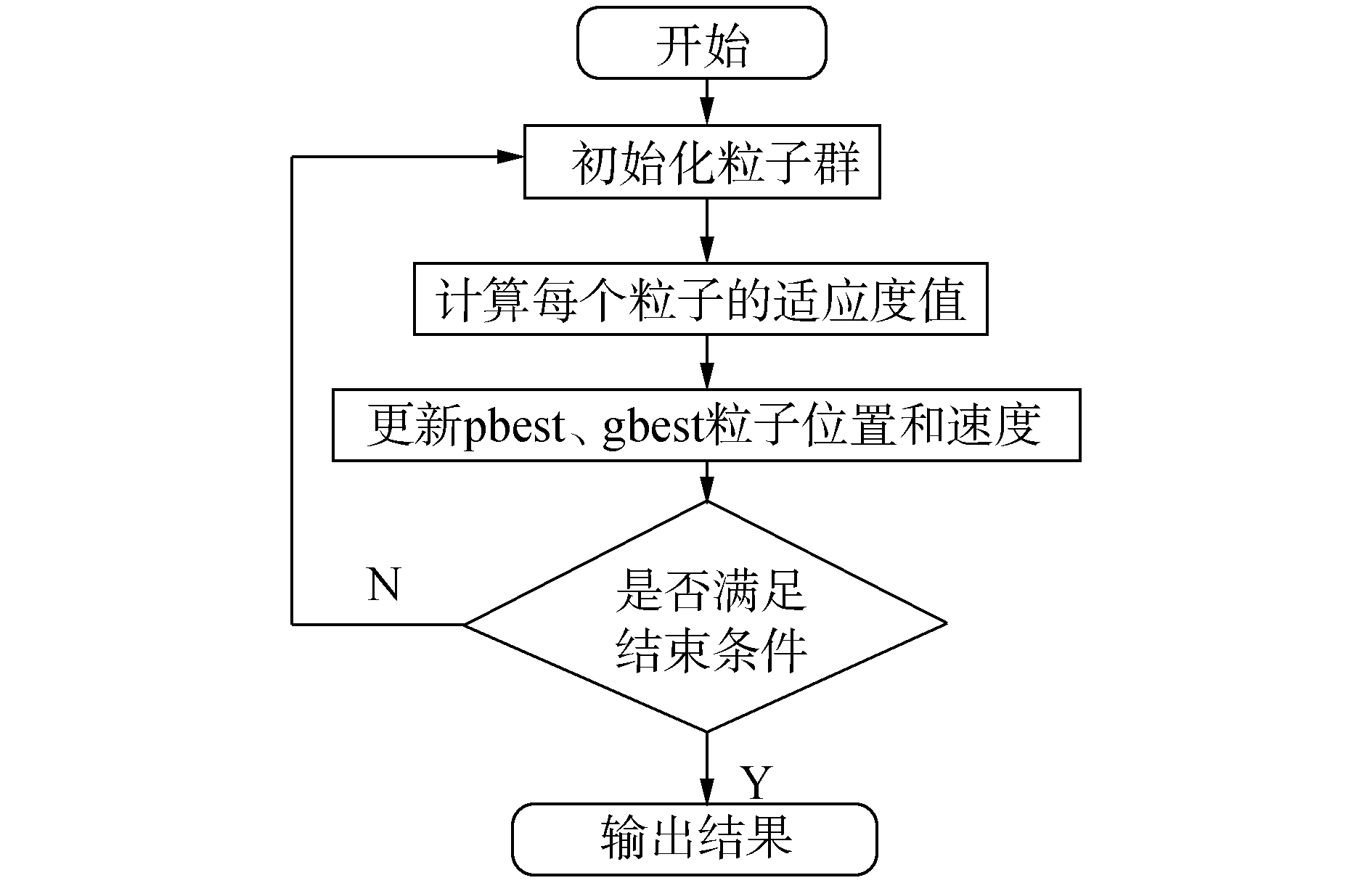图 1 PSO算法流程图 Fig.1 The flowchart of particle swarm optimization algorithm

1.2 粗糙集

 $\begin{array}{l} {\rm{IND}}\left( B \right) = \{ \left( {x,y} \right) \in U \times U\left| {\forall b \in B,} \right.{\rm{ }}\\ b\left( x \right) = b\left( y \right)\} \end{array}$ (1)

 $\underline B X = \{ x{\left| {\left[ x \right]} \right._B} \subseteq X\}$ (2)

 $\overline B X = \{ x{\left| {\left[ x \right]} \right._B} \cap X \ne \emptyset \}$ (3)

 ${\rm{BN}}\left( X \right) = \overline B X - \underline B X$ (4)
2 基于粗糙集相对分类信息熵的粒子群特征选择方法

 $\begin{array}{l} U/B = {X_1} + {X_2} + \ldots + {X_m}\\ U/D = {Y_1} + {Y_2} + \ldots + {Y_k} \end{array}$

 ${p_{ij}} = {\rm{ }}\frac{{\left| {{X_i} \cap {Y_j}} \right|}}{{\left| {{X_i}} \right|}}$ (5)

 $H\left( {U/B} \right) = - \sum\limits_{i = 1}^m {\sum\limits_{j = 1}^k {\frac{{\left| {{X_i}} \right|}}{{\left| U \right|}}{\rm{ }}{p_{ij}}{{\log }_2}{p_{ij}}} }$ (6)

1) 随机初始化大小为N的种群;

2) repeat;

3) for(i=1;iN; i=i+1);

4) 用式(6) 计算种群中每一个个体的适应度值;

5) 更新历史最佳位置best;

6) end for;

7) 更新全局最佳位置gbest;

8) for(i=1;iN; i=i+1);

9) for(d=1;dD; d=d+1);

10) 更新速度信息vid;

11) 更新位置信息xid;

12) end for;

13) end for;

14) until(满足终止条件时);

15) 输出A′。

1) 随机产生N0个0-1向量(粒子)作为初始粒子群, 其中N0是种群的大小。

2) 利用式(6) 计算所有粒子的适应度值。

3) 更新粒子历史最优点pbest：将所有粒子的适应度值和其历史最佳点pbest做比对，择优更新pbest。

4) 更新全局最最优点gbest：将所有粒子的适应度值和全局最优点gbest做比对，择优更新gbest。

5) 根据上一步更新得到的pbest和gbest，更新粒子的速度和位置。其中速度是根据上一步得到的pbest和gbest按照公式(7) 进行更新：

 $\begin{array}{l} v_i^{k + 1} = wv_i^k + {c_1}{\rm{rand}}\left( {{\rm{pbest}} - x_i^k} \right) + \\ {c_2}{\rm{rand}}({\rm{gbest}} - x_i^k) \end{array}$ (7)

 $\begin{array}{l} {x_{ij}} = \left\{ \begin{array}{l} 1,{\rm{rand}}\left( {{v_{i{\rm{ }}j}}} \right) < {\rm{Sigmoid}}\left( {{v_{ij}}} \right)\\ 0,{\rm{其他}} \end{array} \right.\\ {\rm{Sigmoid}}\left( {{v_{ij}}} \right) = \frac{1}{{1 + {{\rm{e}}^{ - {v_{ij}}}}}} \end{array}$ (8)

6) 若迭代次数已达到设定值，终止并输出当前gbest对应的解; 否则，令k=k+1转入2)。

3 实验结果及统计分析表 1 实验结果比较 Tab.1 The comparison of experimental results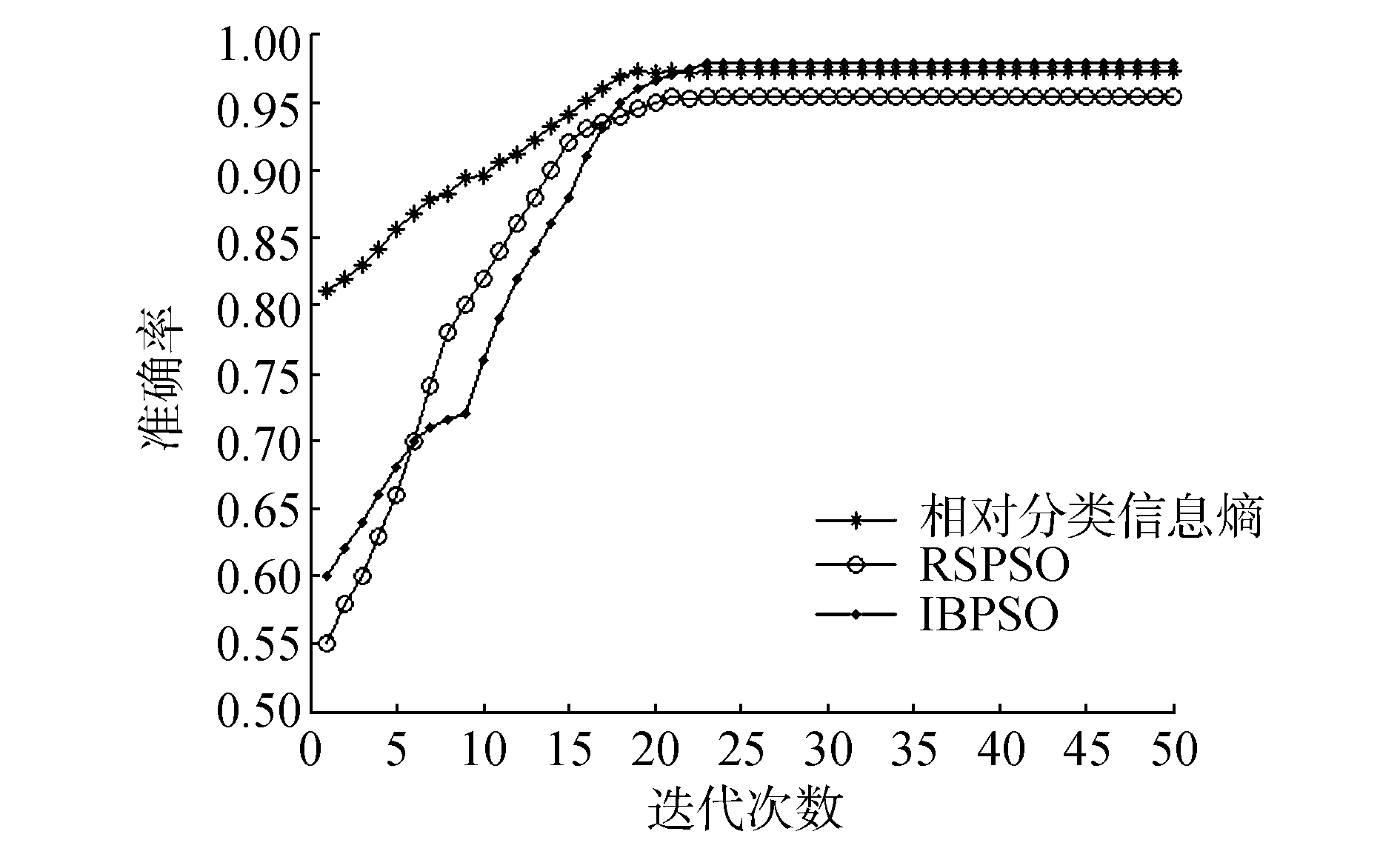图 2 在Kr-vs-Kp数据集上的实验结果 Fig.2 The experimental results of data set Kr-vs-Kp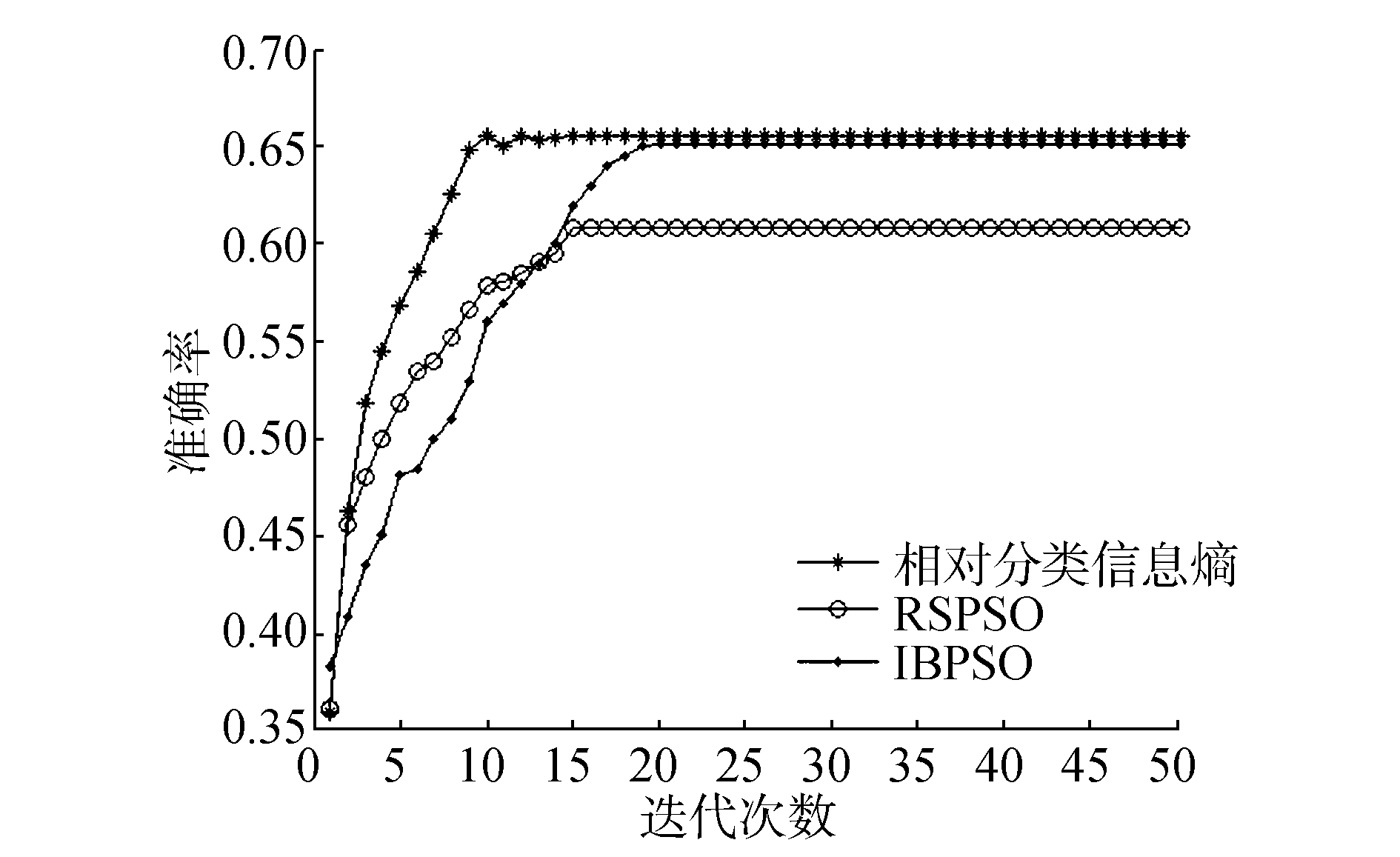图 3 在Voting数据集上的实验结果 Fig.3 The experimental results of data set Voting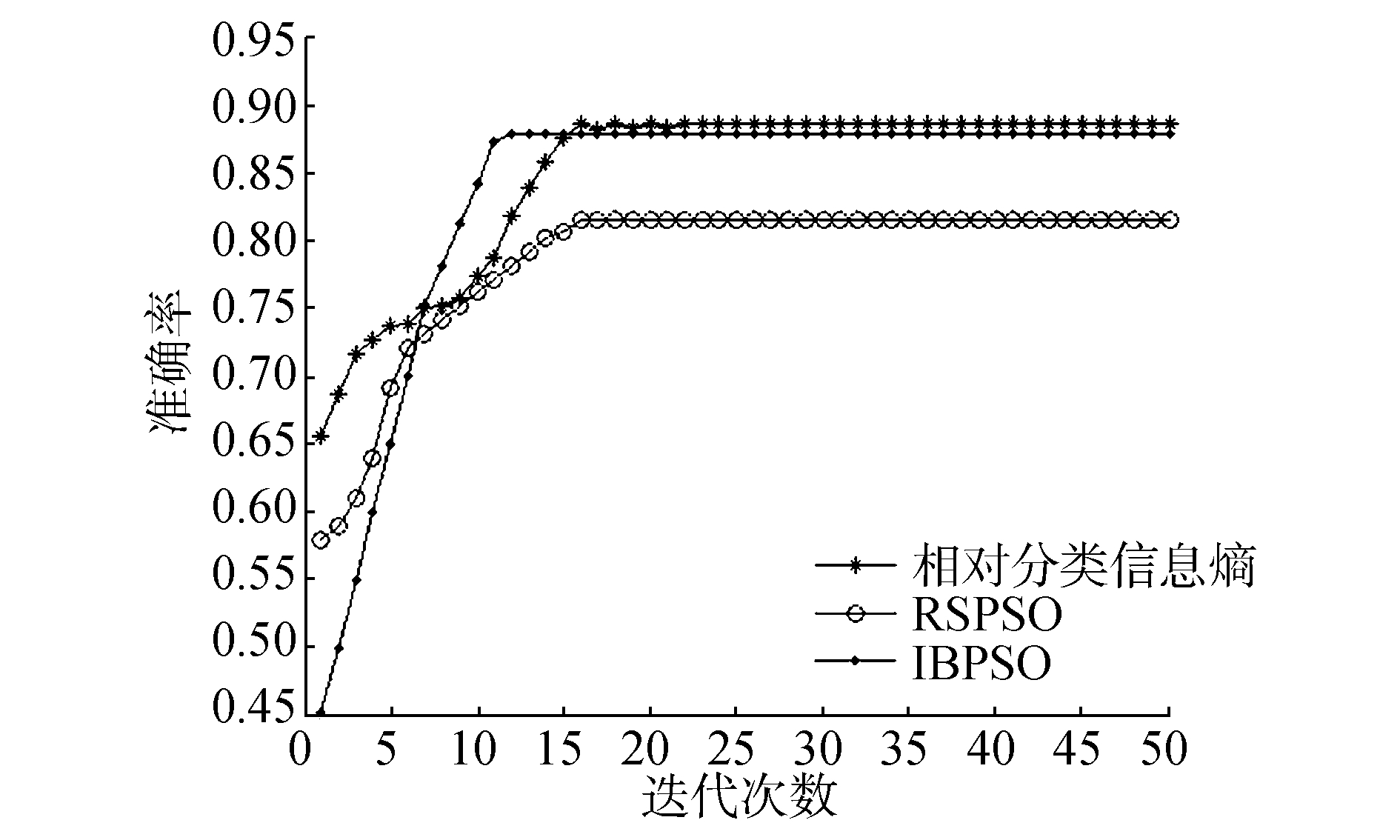图 4 在Mushroom数据集上的实验结果 Fig.4 The experimental results of data set Mushroom图 5 在Nursery数据集上的实验结果 Fig.5 The experimental results of data set Nursery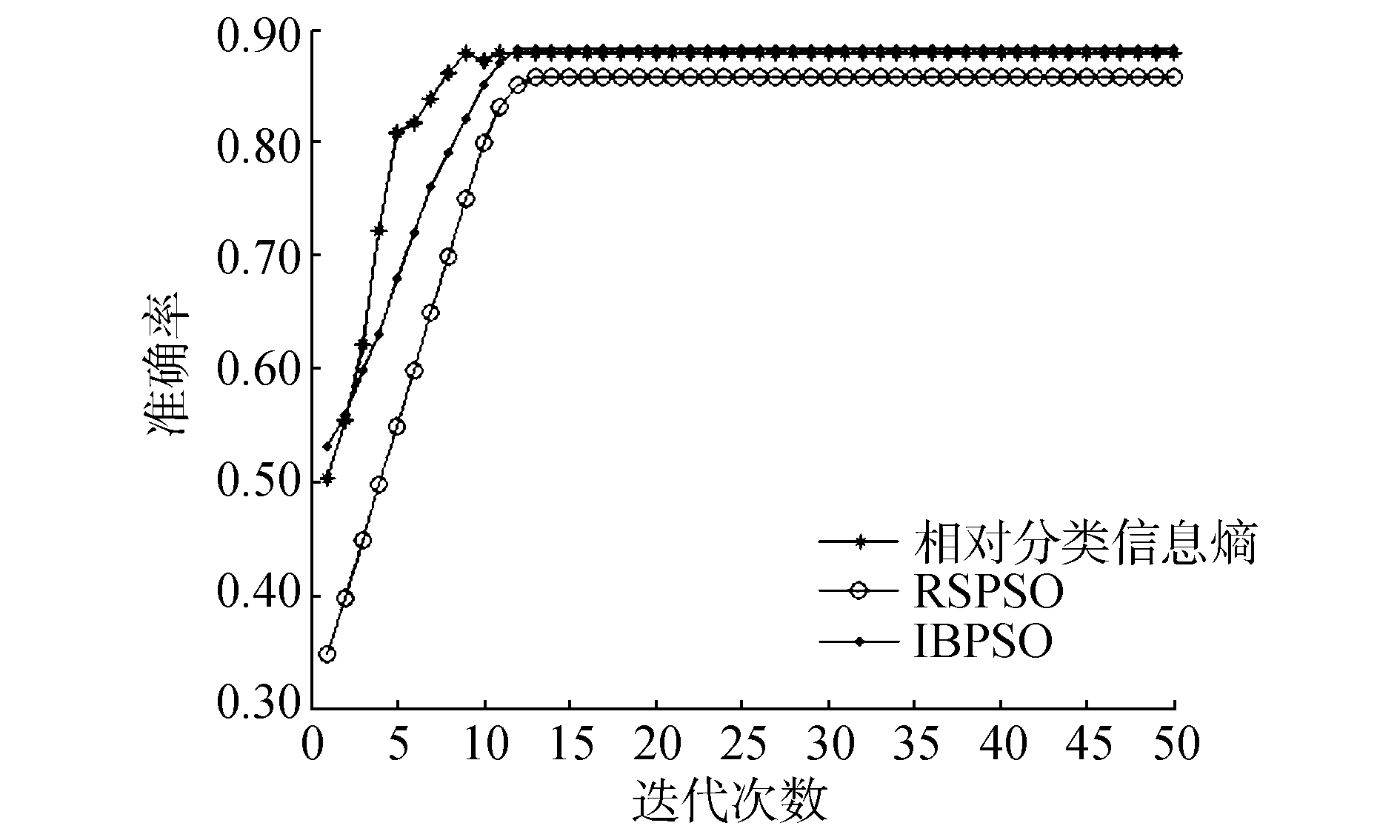图 6 在Car数据集上的实验结果 Fig.6 The experimental results of data set Car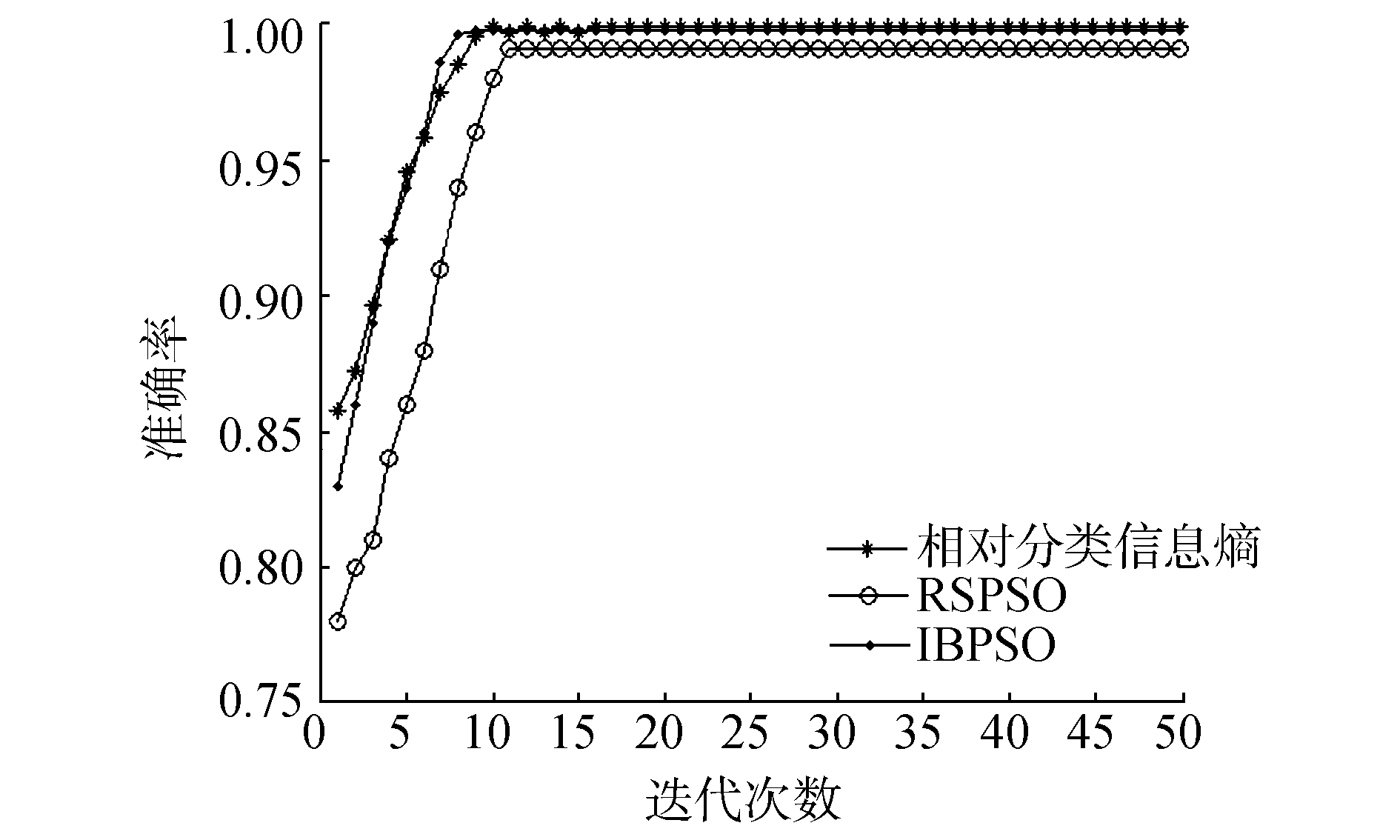图 7 在Soybean数据集上的实验结果 Fig.7 The experimental results of data set Soybean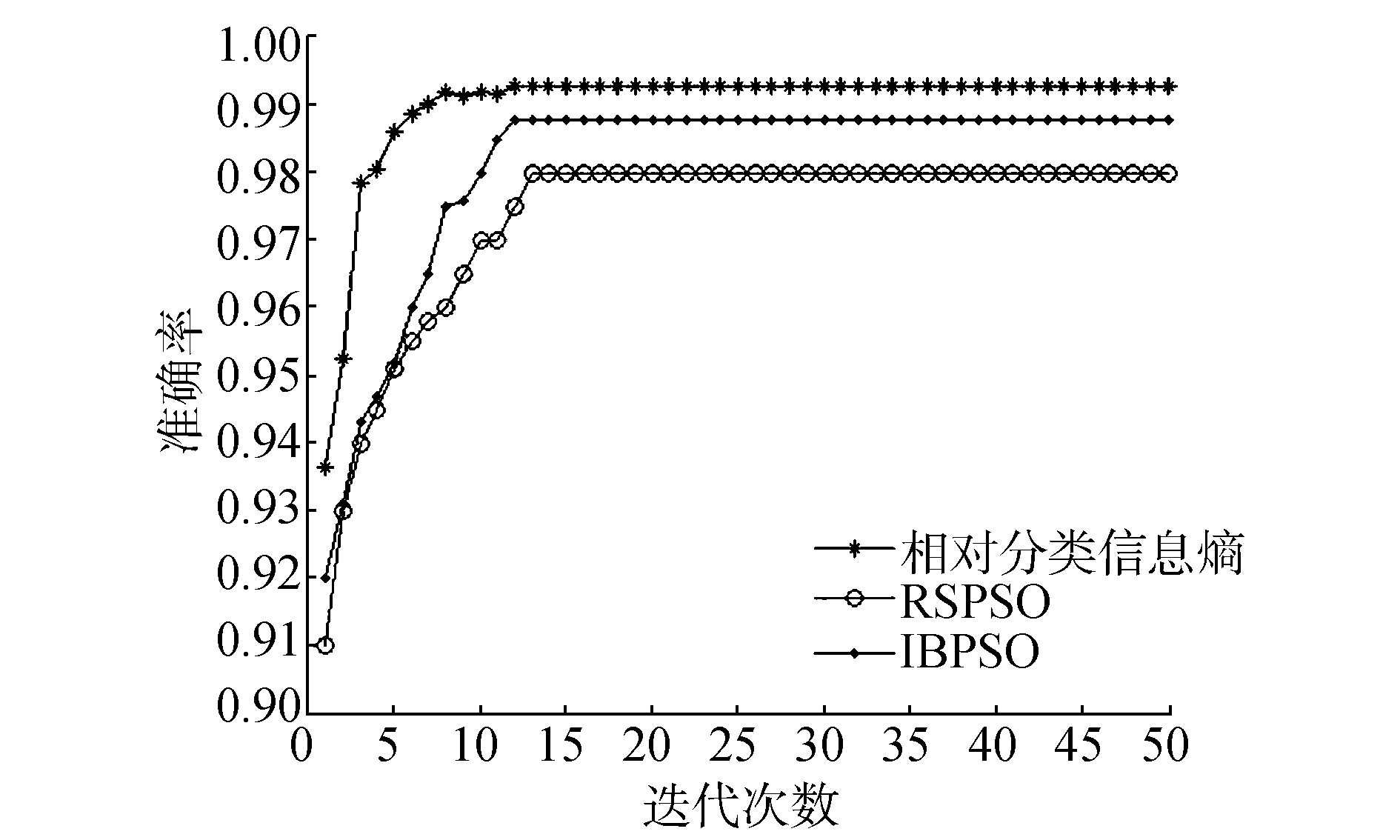图 8 在Tic-Tac-Toe数据集上的实验结果 Fig.8 The experimental results of data set Tic-Tac-Toe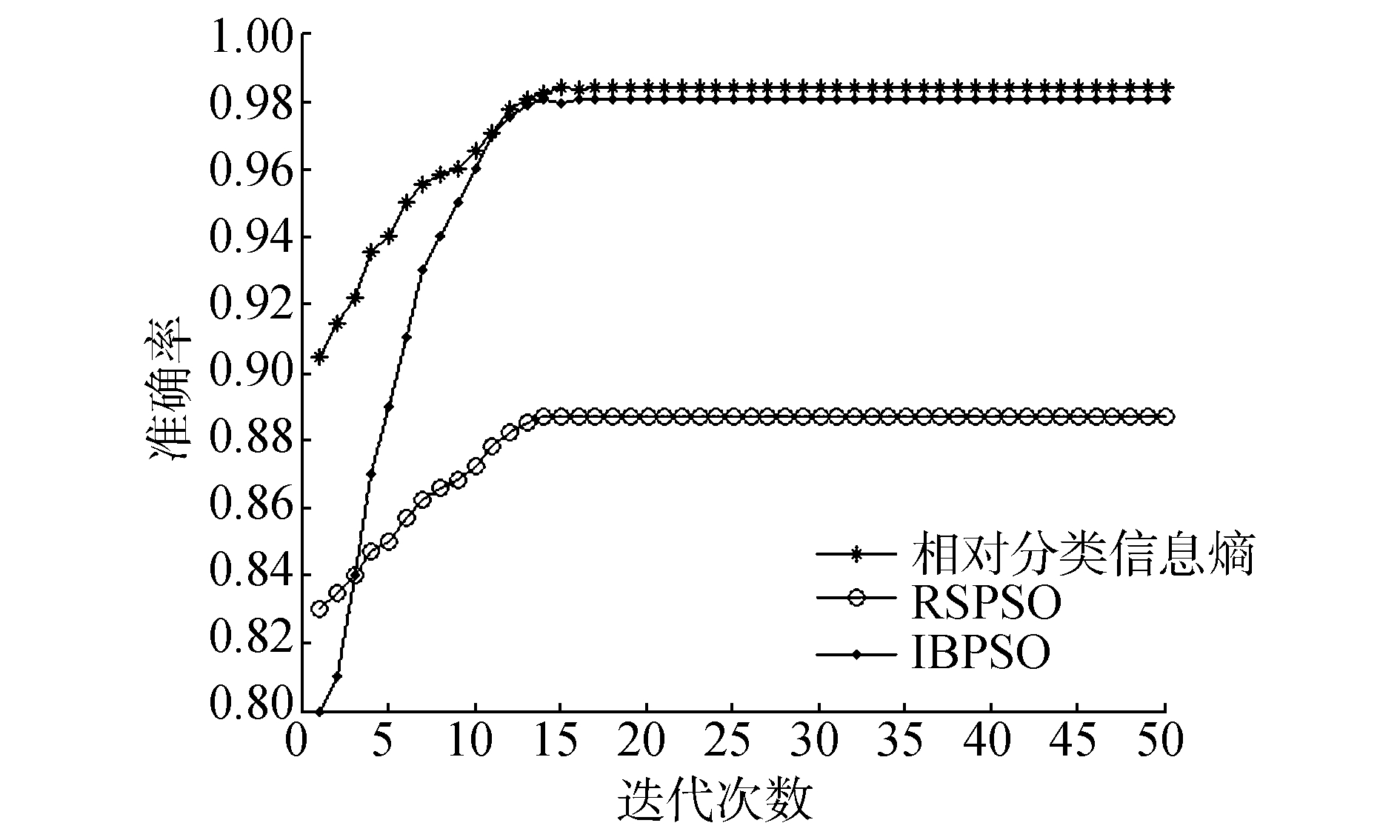图 9 在SPECT数据集上的实验结果 Fig.9 The experimental results of data set SPECT表 2 与遗传算法比较的实验结果(1) Tab.2 The experimental results compared with genetic algorithm(1)表 3 与遗传算法比较的实验结果(2) Tab.3 The experimental results compared with genetic algorithm(2)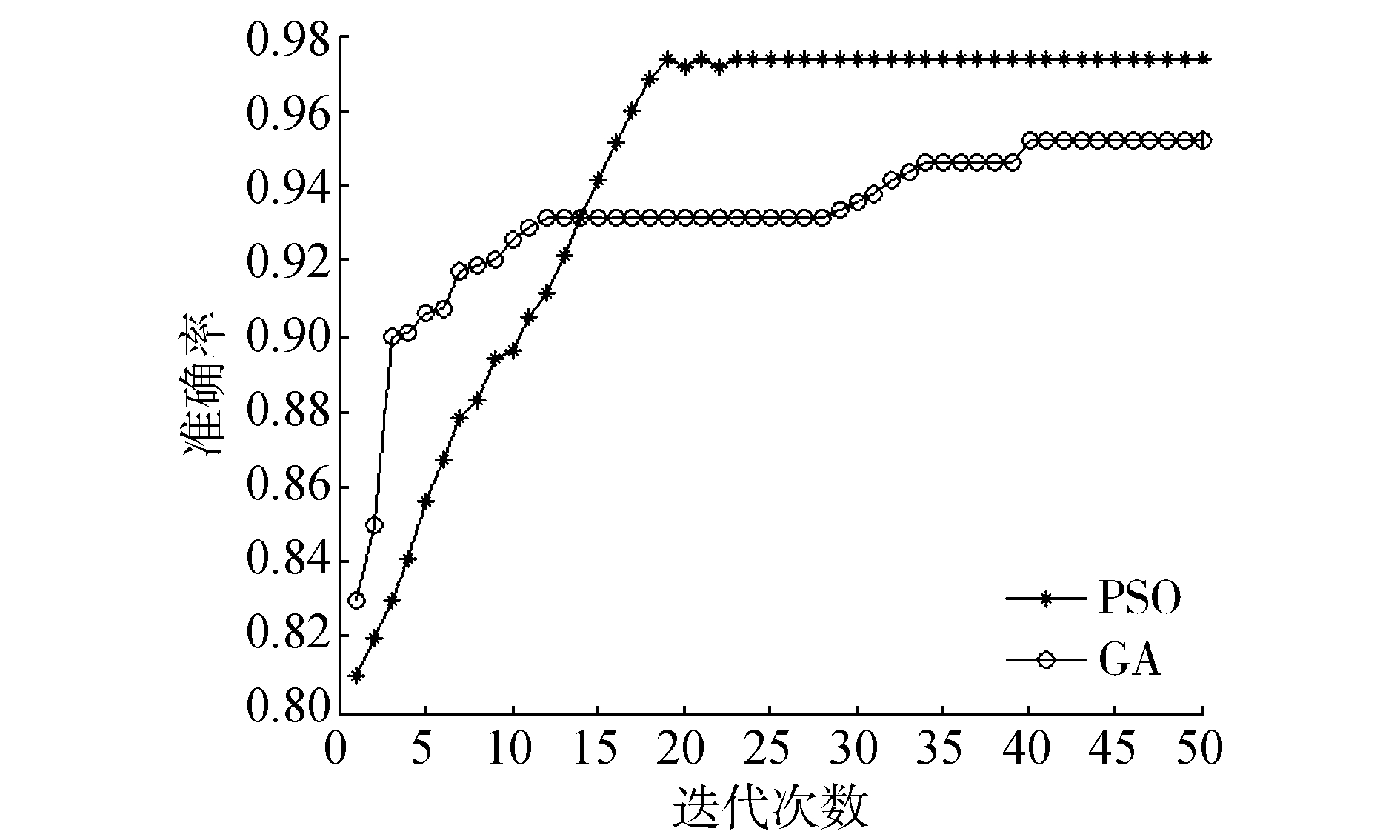图 10 在数据集Kr-vs-Kp上的实验结果(PSO与GA对比) Fig.10 The experimental results of data set Kr-vs-Kp (PSO, GA)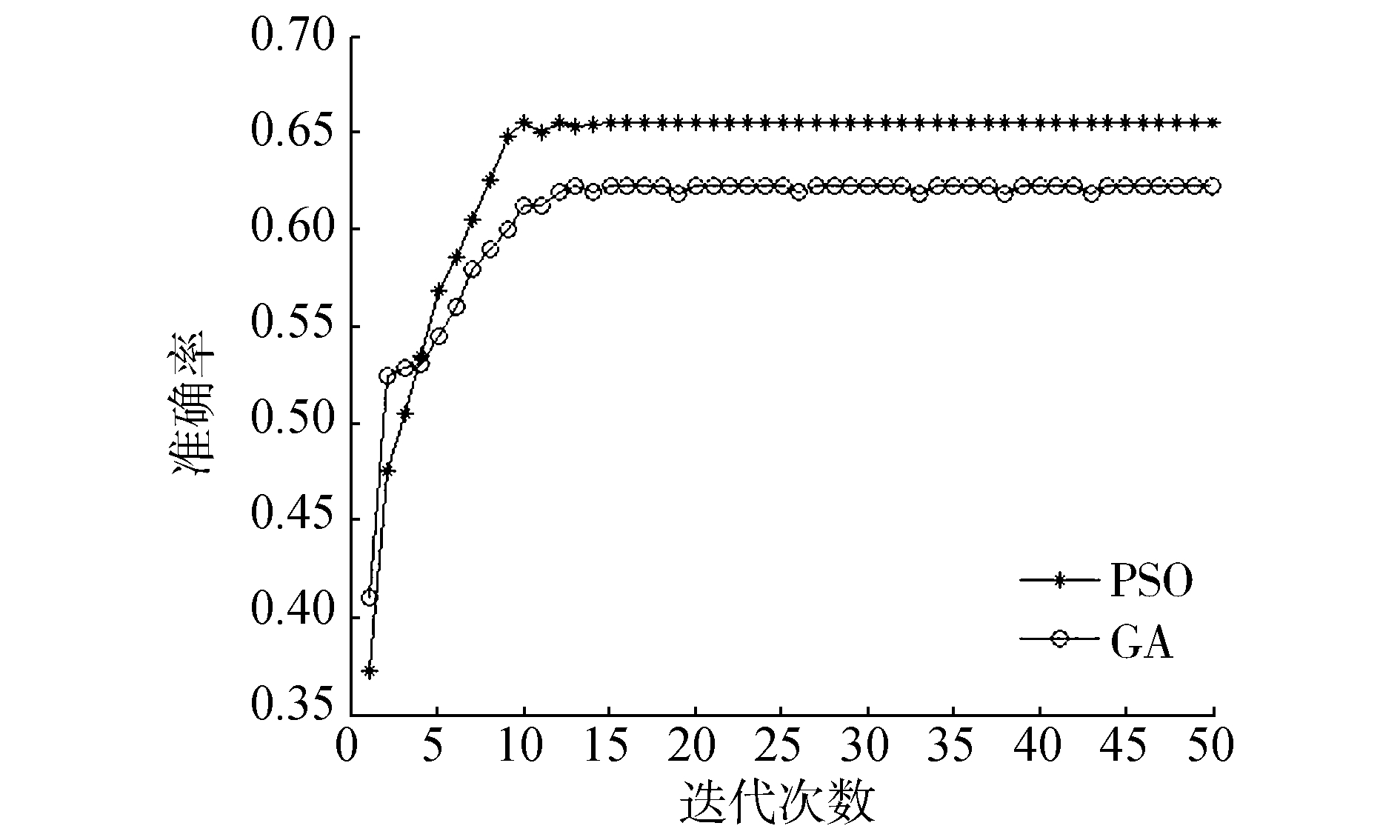图 11 在数据集Voting上的实验结果(PSO与GA对比) Fig.11 The experimental results of data set Voting(PSO, GA)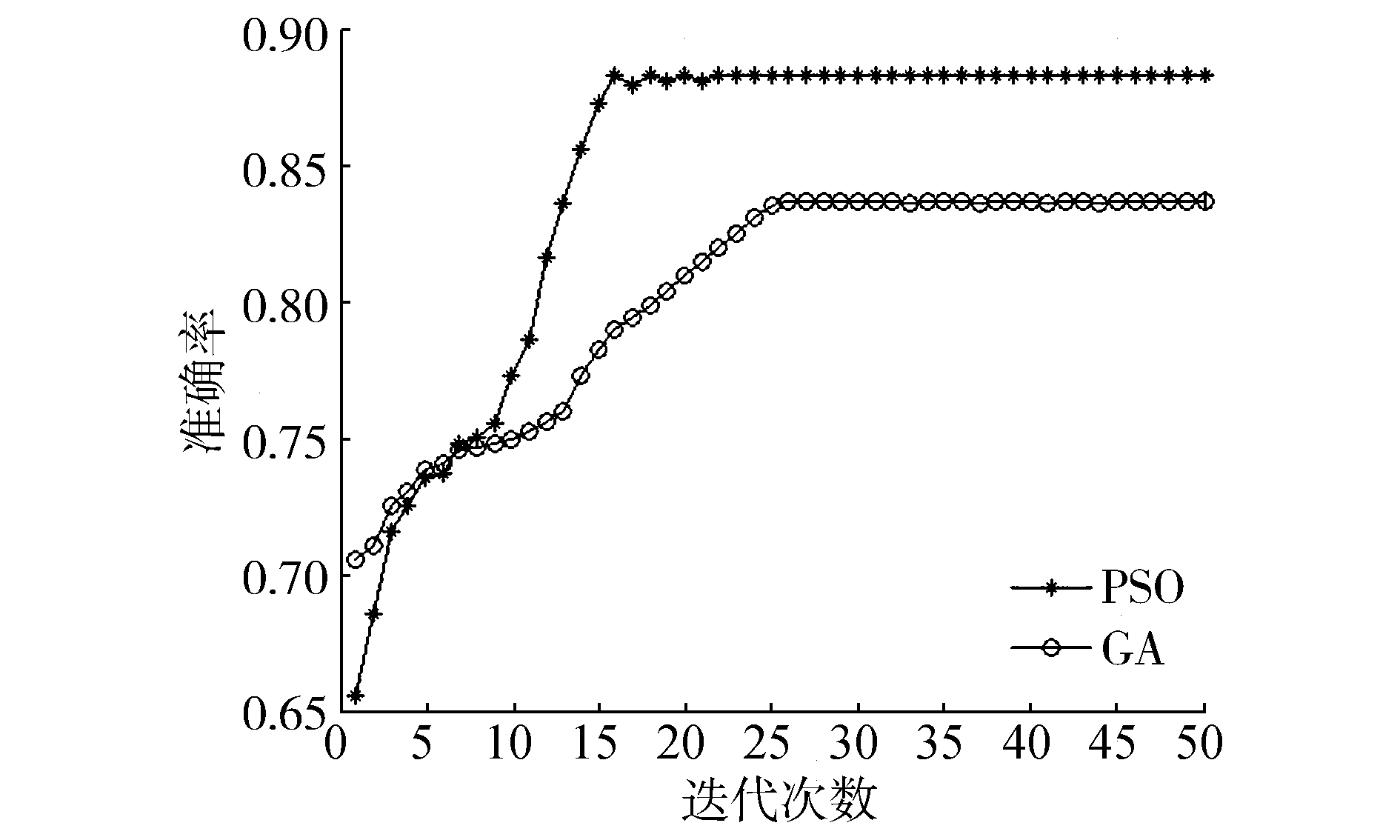图 12 在数据集Mushroom上的实验结果(PSO与GA对比) Fig.12 The experimental results of data set Mushroom(PSO, GA)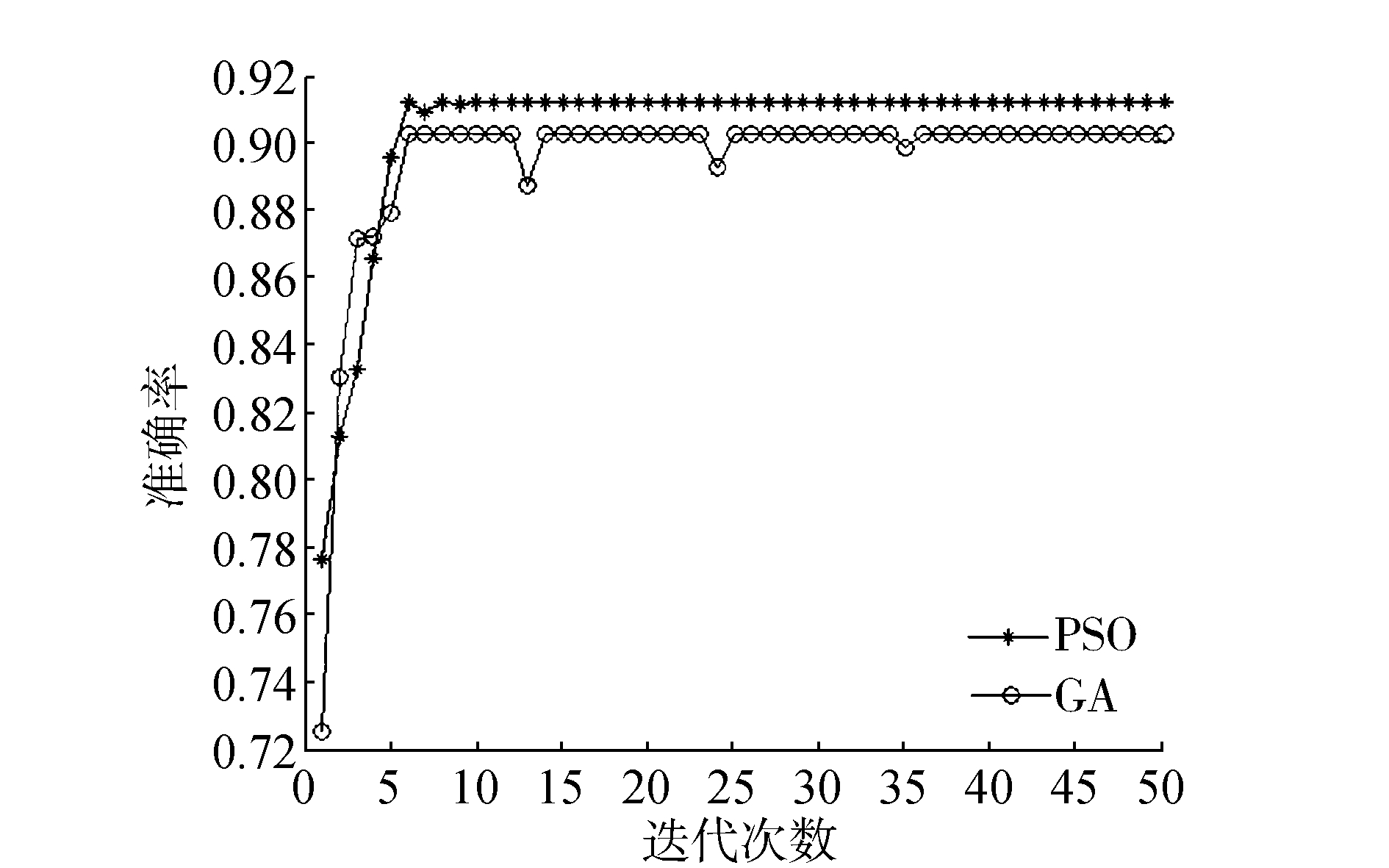图 13 在数据集Nursery上的实验结果(PSO与GA对比) Fig.13 The experimental results of data set Nursery(PSO, GA)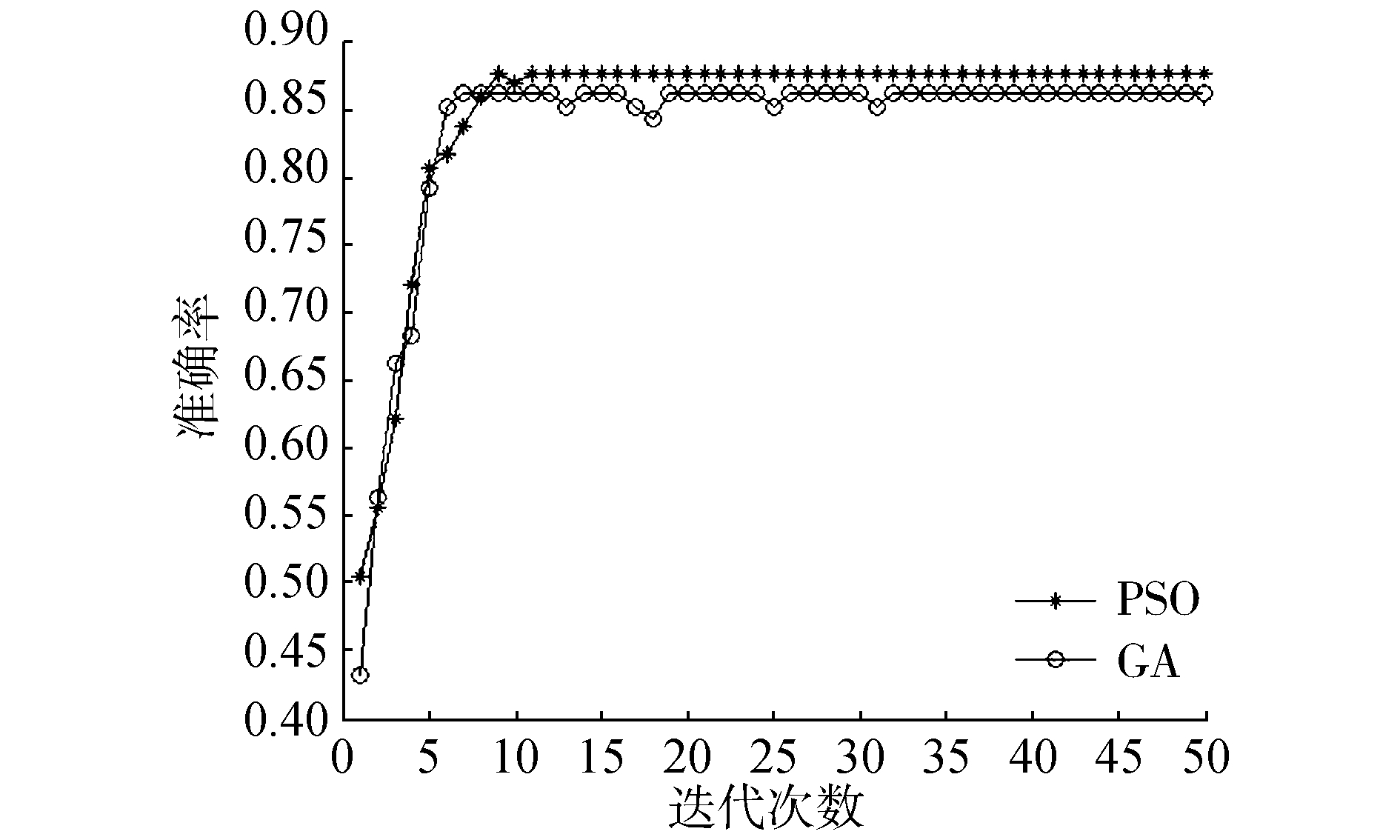图 14 在数据集Car上的实验结果(PSO与GA对比) Fig.14 The experimental results of data set Car(PSO, GA)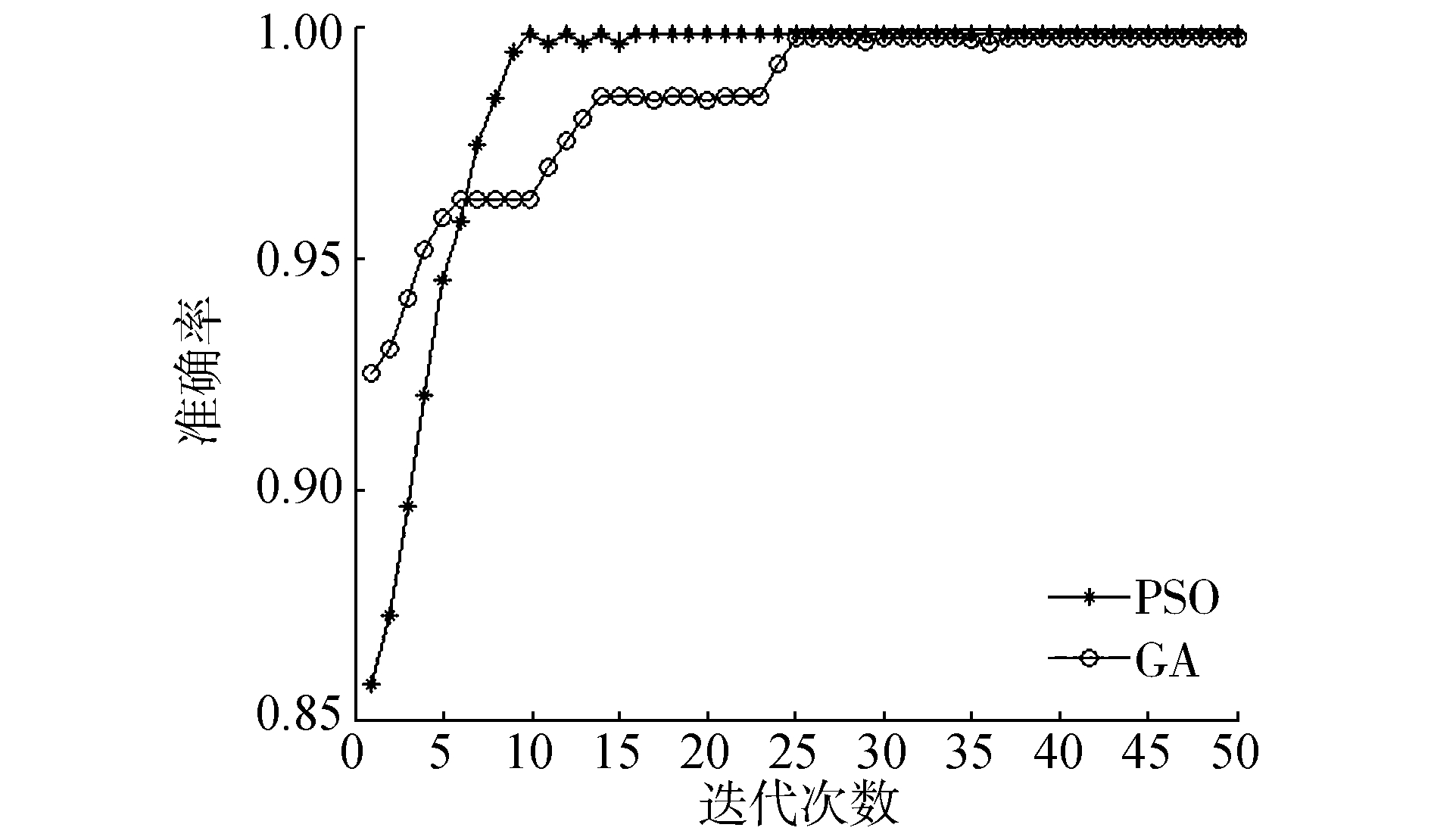图 15 在数据集Soybean上的实验结果(PSO与GA对比) Fig.15 The experimental results of data set Soybean(PSO, GA)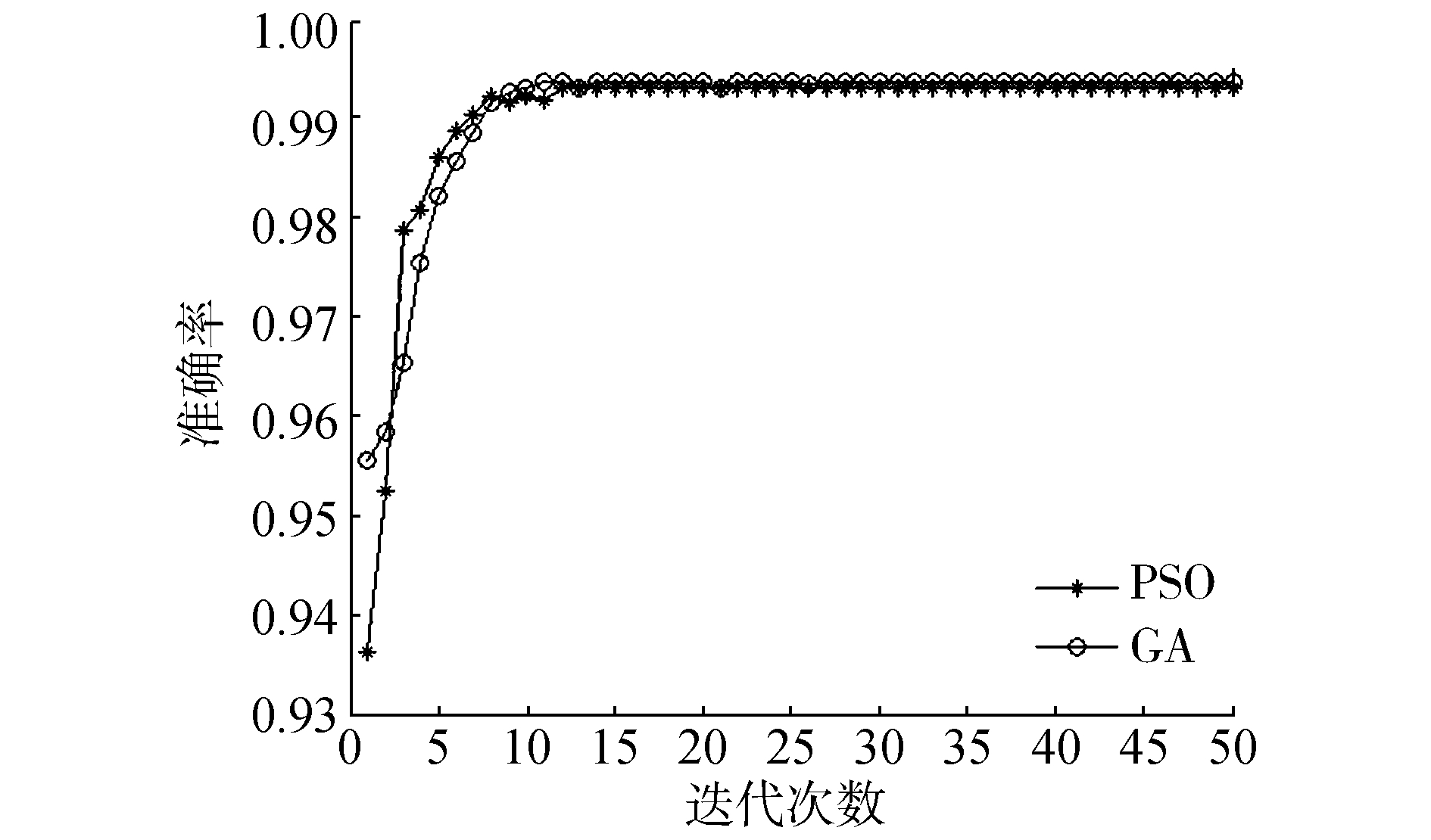图 16 在数据集Tic-Tac-Toe上的实验结果(PSO与GA对比) Fig.16 The experimental results of data set Tic-Tac-Toe(PSO, GA)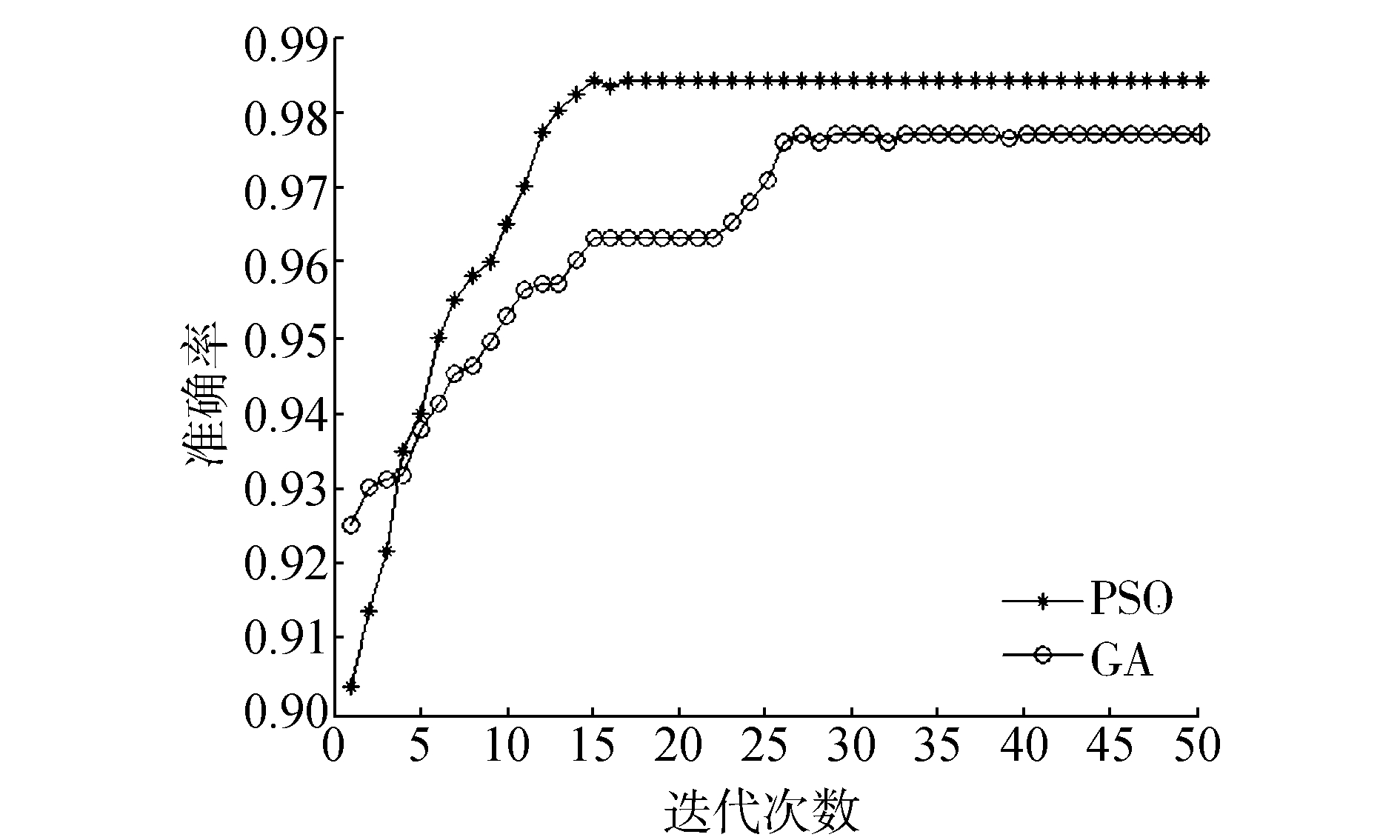图 17 在数据集SPECT上的实验结果(PSO与GA对比) Fig.17 The experimental results of data set SPECT(PSO, GA)表 4 配对t检验p Tab.4 p values of paired t-test

p值均较小，显著性差异较大，进一步证明了方法的可行。

4 结论

  GUYON I, GUNN S, NIKRAVESH M, et al. Feature extraction, foundations and applications[M]. Berlin: Springer, 2006. (0)  DASH M, LIU H. Feature selection for classification[J]. Intelligent data analysis, 1997, 1: 131-151. DOI:10.1016/S1088-467X(97)00008-5 (0)  PAWLAK Z. Rough sets[J]. Internationa journal of information and computer sciences, 1982, 11: 341-356. DOI:10.1007/BF01001956 (0)  苗夺谦, 李道国. 粗糙集理论、算法与应用[M]. 北京: 清华大学出版社, 2008. (0)  SWINIARSKI R W, SKOWRON A. Rough set methods in feature selection and recognition[J]. Pattern recognition letters, 2003, 24(6): 833-849. DOI:10.1016/S0167-8655(02)00196-4 (0)  JENSEN R, SHEN Q. Fuzzy-rough sets for descriptive dimensionality reduction[C]//IEEE International Conference on Fuzzy Systems, 2002. Fuzz-IEEE. 2002:29-34. (0)  BHATT R B, GOPAL M. On fuzzy-rough sets approach to feature selection[J]. Pattern recognition letters, 2005, 26(3): 965-975. DOI:10.1016/j.patrec.2004.09.044 (0)  JENSEN R, PARTHALáIN N M. Towards scalable fuzzy rough feature selection[J]. Information sciences, 2015, 323(C): 1-15. (0)  QIAN Y H, LIANG J, PEDRYCZ W, et al. Positive approximation: an accelerator for attribute reduction in rough set theory[J]. Artificial intelligence, 2010, 174(9/10): 597-618. (0)  HU Q H, YU D R, LIU J F, et al. Neighborhood rough set based heterogeneous feature subset selection[J]. Information sciences, 2008, 178(18): 3577-3594. DOI:10.1016/j.ins.2008.05.024 (0)  ALMUALLIM H, DIETTERICH T G. Learning boolean concepts in the presence of many irrelevant features[J]. Artificial intelligence, 1994, 69(1/2): 279-305. (0)  DASH M, LIU H. Consistency-based search in feature selection[J]. Artificial intelligence, 2003(151): 155-176. (0)  BATTITI R. Using mutual information for selecting features in supervised neural net learning[J]. IEEE transactions on neural networks, 1994, 5(4): 537-549. DOI:10.1109/72.298224 (0)  KWAK N, CHOI C H. Input feature selection by mutual information based on parzen window[J]. IEEE transactions on pattern analysis and machine intelligence, 2002, 24(12): 1667-1671. DOI:10.1109/TPAMI.2002.1114861 (0)  ESTEVEZ P A, TESMER M, PEREZ C A, et al. Normalized mutual information feature selection[J]. IEEE transactions on neural networks, 2009, 20(2): 189-201. DOI:10.1109/TNN.2008.2005601 (0)  SONG L, SMOLA A, GRETTON A, et al. Feature selection via dependence maximization[J]. Journal of machine learning research, 2012, 13: 1393-1434. (0)  HU Q H, ZHU Pengfei, LIU Jinfu, et al. Feature selection via maximizing fuzzy dependency[J]. Fundamenta informaticae, 2010, 98: 167-181. (0)  KOHAVI R, JOHN G. Wrappers for feature subset selection[J]. Artificial intelligence, 1997, 97(1/2): 273-324. (0)  SINDHWANI V, RAKSHIT S, DEODHARE D, et al. Feature selection in MLPs and SVMs based on maximum output information[J]. IEEE transactions on neural networks, 2004, 15(4): 937-947. DOI:10.1109/TNN.2004.828772 (0)  YANG Jianbo, SHEN Kaiquan, ONG Chongjin, et al. Feature selection for MLP neural network: the use of random permutation of probabilistic outputs[J]. IEEE transactions on neural networks, 2009, 20(12): 1911-1922. DOI:10.1109/TNN.2009.2032543 (0)  QUINLAN J R. Induction of decision trees[J]. Machine learning, 1986, 1: 81-106. (0)  BREIMAN L, FRIEDMAN J H, RICHARD A S, et al. Classification and regression trees[M]. Belmont, CA: wadsworth international group, 1984. (0)  SETIONO R, LIU H. Neural-network feature selector[J]. IEEE transactions on neural networks, 1997, 8(3): 654-662. DOI:10.1109/72.572104 (0)  SHEN Kaiquan, ONG Chongjin, LI Xiaoping, et al. Feature selection via sensitivity analysis of SVM probabilistic outputs[J]. Machine learning, 2008, 70: 1-20. (0)  PERKINS S, LACKER K, THEILER J. Grafting: fast, incremental feature selection by gradient descent in function space[J]. Journal of machine learning research, 2003(3): 1333-1356. (0)  KENNEDY J, EBERHART R. Particle swarm optimization [C]. IEEE International Conference on Neural Networks. Perth, Australia, 1995, 4: 1942-1948. (0)  EBERHART R C, SHI Y H, KENNEDY J. Swarm Intelligence[M]. Massachusetts: Morgan Kaufmann, 2001. (0)  EBERHART R C, KENNEDY J. A discrete binary version of the particle swarm algorithm[J]. IEEE conference on systems, 1997, 5: 4104-4109. (0)  CHUANG L Y, CHANG H W, TU C J, et al. Improved binary PSO for feature selection using gene expression data[J]. Computational biology & chemistry, 2008, 32(1): 29-37. (0)  CHUANG L Y, TSAI S W, YANG C H. Improved binary particle swarm optimization using catfish effect for feature selection[J]. Expert systems with applications, 2011, 38(10): 12699-12707. DOI:10.1016/j.eswa.2011.04.057 (0)  WANG Xiangyang, YANG Jie, TENG Xiaolong, et al. Feature selection based on rough sets and particle swarm optimization[J]. Pattern recognition letters, 2007, 28(4): 459-471. DOI:10.1016/j.patrec.2006.09.003 (0)  CERVANTE L, XUE B, ZHANG M, et al. Binary particle swarm optimisation for feature selection: a filter based approach[J]. Evolutionary computation, 2012, 41: 1-8. (0)  LIU Quanjin, ZHAO Zhimin, LI Yingxin. Ensemble feature selection method based on neighborhood information and PSO algorithm[J]. Acta electronica sinica, 2016, 44(4): 995-1002. (0)  FONG S, WONG R, VASILAKOS A. Accelerated PSO swarm search feature selection for data stream mining big data[J]. IEEE transactions on services computing, 2016, 9(1): 33-45. (0)  翟俊海, 刘博, 张素芳. 基于相对分类信息熵的进化特征选择算法[J]. 模式识别与人工智能, 2016, 29(8): 682-690. ZHAI Junhai, LIU Bo, ZHANG Sufang. Feature selection via evolutionary computation based on relative classification information entropy[J]. Pattern recognition and artificial intelligence, 2016, 29(8): 682-690. (0)  SHI B Y, EBERHART R. A modified particle swarm optimizer[J]. IEEE world congress on computational intelligence, 1999, 6: 69-73. (0)